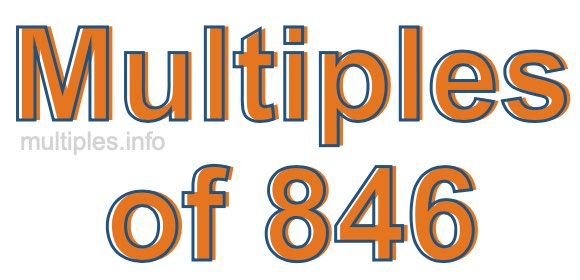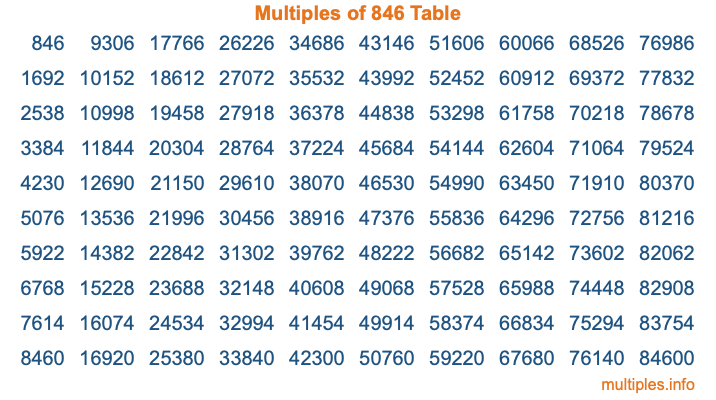Multiples of 846Welcome to the Multiples of 846 page. Here we will first teach you everything you will ever need to know about the multiples of 846, and then give you a study guide summary of everything we taught you to make sure you remember it all. Use this page to look up facts and learn information about the multiples of 846. This page will make you a multiples of eight hundred forty-six expert!

Definition of Multiples of 846
Multiples of 846 are all the numbers that when divided by 846 equal an integer. Each of the multiples of 846 are called a multiple. A multiple of 846 is created by multiplying 846 by an integer.

Therefore, to create a list of multiples of 846, you start with 1 multiplied by 846, then 2 multiplied by 846, then 3 multiplied by 846, and so on for as long as you want. Thus, the list of the first five multiples of 846 is 846, 1692, 2538, 3384, and 4230. To see a larger list of multiples of 846, see the printable image of Multiples of 846 further down on this page. We also have a category where you can choose any nth multiple of 846.

Multiples of 846 Checker
The Multiples of 846 Checker below checks to see if any number of your choice is a multiple of 846. In other words, it checks to see if there is any number (integer) that when multiplied by 846 will equal your number. To do that, we divide your number by 846. If the the quotient is an integer, then your number is a multiple of 846.

Is  a multiple of 846?

Least Common Multiple of 846 and ...
A Least Common Multiple (LCM) is the lowest multiple that two or more numbers have in common. This is also called the smallest common multiple or lowest common multiple and is useful to know when you are adding our subtracting fractions. Enter one or more numbers below (846 is already entered) to find the LCM.

Check out our LCM Calculator if you need more details about the Least Common Multiple or if you need the LCM for different numbers for adding and subtraction fractions.

nth Multiple of 846
As we stated above, 846 is the first multiple of 846, 1692 is the second multiple of 846, 2538 is the third multiple of 846, and so on. Enter a number below to find the nth multiple of 846.

th multiple of 846

Multiples of 846 vs Factors of 846
846 is a multiple of 846 and a factor of 846, but that is where the similarities end. All postive multiples of 846 are 846 or greater than 846. All positive factors of 846 are 846 or less than 846.

Below is the beginning list of multiples of 846 and the factors of 846 so you can compare:

Multiples of 846: 846, 1692, 2538, 3384, 4230, etc.

Factors of 846: 1, 2, 3, 6, 9, 18, 47, 94, 141, 282, 423, 846

As you can see, the multiples of 846 are all the numbers that you can divide by 846 to get a whole number. The factors of 846, on the other hand, are all the whole numbers that you can multiply by another whole number to get 846.

It's also interesting to note that if a number (x) is a factor of 846, then 846 will also be a multiple of that number (x).

Multiples of 846 vs Divisors of 846
The divisors of 846 are all the integers that 846 can be divided by evenly. Below is a list of the divisors of 846.

Divisors of 846: 1, 2, 3, 6, 9, 18, 47, 94, 141, 282, 423, 846

The interesting thing to note here is that if you take any multiple of 846 and divide it by a divisor of 846, you will see that the quotient is an integer.

Multiples of 846 Table
Below is an image of the first 100 multiples of 846 in a table. The table is in chronological order, column by column. The first column has the first ten multiples of 846, the second column has the next ten multiples of 846, and so on.The Multiples of 846 Table is also referred to as the 846 Times Table or Times Table of 846. You are welcome to print out our table for your studies.

Negative Multiples of 846
Although not often discussed or needed in math, it is worth mentioning that you can make a list of negative multiples of 846 by multiplying 846 by -1, then by -2, then by -3, and so on, to get the following list of negative multiples of 846:

-846, -1692, -2538, -3384, -4230, etc.

Multiples of 846 Summary
Below is a summary of important Multiples of 846 facts that we have discussed on this page. To retain the knowledge on this page, we recommend that you read through the summary and explain to yourself or a study partner why they hold true.

There are an infinite number of multiples of 846.

A multiple of 846 divided by 846 will equal a whole number.

846 divided by a factor of 846 equals a divisor of 846.

The nth multiple of 846 is n times 846.

The largest factor of 846 is equal to the first positive multiple of 846.

846 is a multiple of every factor of 846.

846 is a multiple of 846.

A multiple of 846 divided by a divisor of 846 equals an integer.

846 divided by a divisor of 846 equals a factor of 846.

Any integer times 846 will equal a multiple of 846.

Multiples of a Number
Here you can get the multiples of another number, all with the same attention to detail as we did for multiples of 846 on this page.

Multiples of
Multiples of 847
Did you find our page about multiples of eight hundred forty-six educational? Do you want more knowledge? Check out the multiples of the next number on our list!